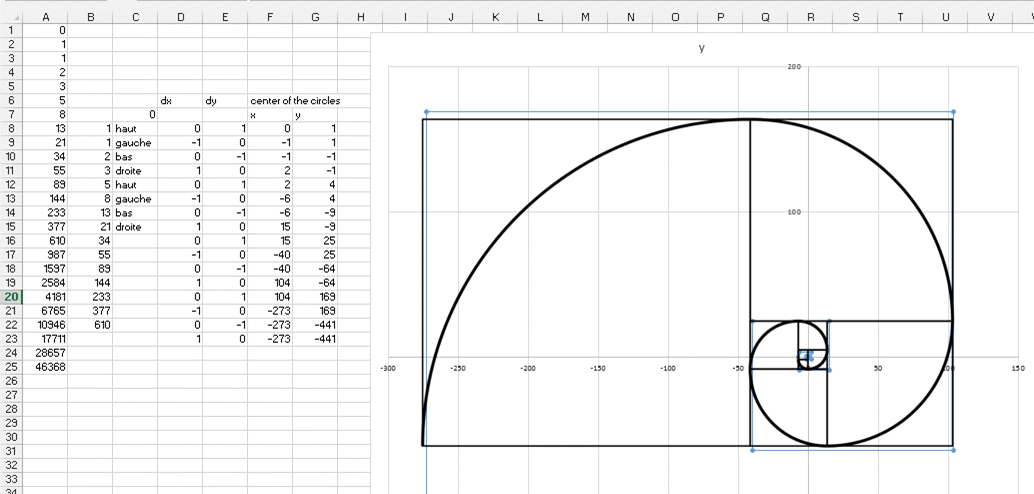## Fibonacci Sequence: a serie created by nature

The Fibonacci Sequence is found in the nature and in movies like the Da Vinci Code.

The first thing is to enter the two first numbers of it, which are 0 and 1.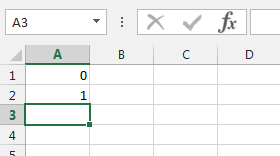Then the series is made like this. The item n equal to the sum of the item N-1 and the item N-2 (or basically the sum of the 2 previous items)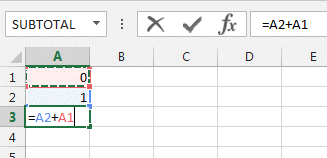Then select the cell 3 and slide it to the bottom.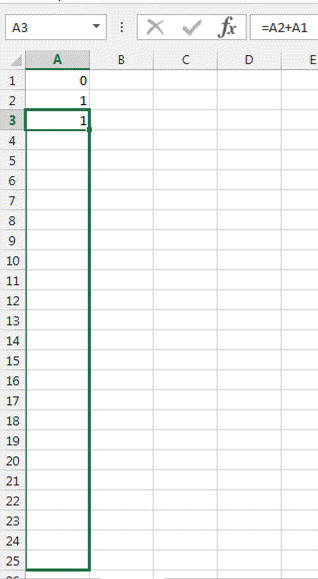And here is the Fibonacci sequence.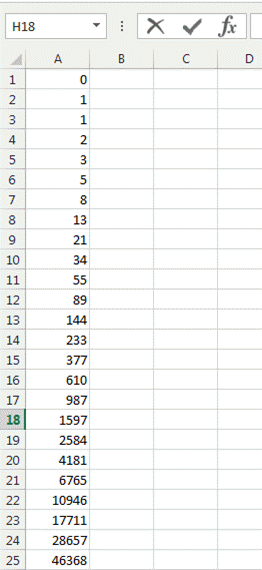Here a graphical representation a the spiral that can be generated. Only the circle center are drawn on a scatter chart. You could use these and then add the circle.

We just superpose the spiral to check the correctness.